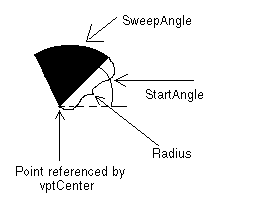﻿ VECTORARC | Used Structures | Vector C++ Class Library Help
In This Topic ▼

# VECTORARC

````typedef struct tagVECTORARC `
`{ `
`   VECTOROBJECT Object; `
`   VECTORPOINT Point; `
`   VECTORPEN Pen; `
`   L_DOUBLE Radius; `
`   L_DOUBLE StartAngle; `
`   L_DOUBLE SweepAngle; `
`} VECTORARC, * pVECTORARC; ````

The VECTORARC structure holds information about a vector arc object.

## Members

Abstract object.

### VECTORPOINT Point

A VECTORPOINT structure that contains the vertex of a vector arc object, about which the arc sweeps.

### VECTORPEN Pen

VECTORPEN structure that contains information about the pen to use when drawing the vector arc object.

Length of the radius of the vector arc object. This value is in logical units.

### L_DOUBLE StartAngle

The angle at which to begin the vector arc. Values should be between 0 and 360 degrees. Values less than 0 and greater than 360 will be modified internally.

### L_DOUBLE SweepAngle

The angle through which to sweep the vector arc. Values should be between 360 and +360 degrees. Positive angles will be drawn in a counter-clockwise direction from StartAngle. Negative angles will be drawn in a clockwise direction.